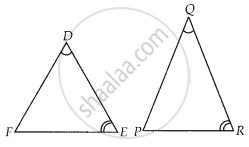# If in two triangles DEF and PQR, ∠D = ∠Q and ∠R = ∠E, then which of the following is not true? - Mathematics

MCQ

If in two triangles DEF and PQR, ∠D = ∠Q and ∠R = ∠E, then which of the following is not true?

#### Options

• (EF)/(PR) = (DF)/(PQ)

• (DE)/(PQ) = (EF)/(RP)

• (DE)/(QR) = (DF)/(PQ)

• (EF)/(RP) = (DE)/(QR)

#### Solution

(DE)/(PQ) = (EF)/(RP)

Explanation:

Given, in ∆DEF and ∆PQR, ∠D = ∠Q, ∠R = ∠E∴ ∆DEF ~ ∆QRP ......[By AAA similarity criterion]

⇒ ∠F = ∠P   .....[Corresponding angles of similar triangles]

∴ (DE)/(QP) = (ED)/(RQ) = (FE)/(PR)

Concept: Similarity of Triangles
Is there an error in this question or solution?

#### APPEARS IN

NCERT Mathematics Exemplar Class 10
Chapter 6 Triangles
Exercise 6.1 | Q 6 | Page 61
Share